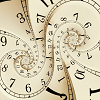#### You may also like### Arithmagons Poster

Arithmagons Poster### Decimal Time Poster

Poster based on Decimal Time task.# Got it Poster

##### Age 7 to 14Challenge Level

You can always win, if you make sure you say the numbers 3, 8, 13 and 18.

Why?
If your opponent says 19, 20, 21 or 22, then you can say 4, 3, 2 or 1 and you win. And if you say 18, then your opponent has to add 1, 2, 3 or 4, so they have to say 19, 20, 21 or 22.

So 18 is a "key" number - you can always win if you say 18.

For the same reason, 13 is a "key" number: if you say 13, your opponent has to say 14, 15, 16 or 17. So you can choose a number between 1 and 4 which will make 18. Once you have said 18, you can win!

Counting backwards, 8 and 3 are also key numbers.

If you have a different target number, there will still be "key" numbers every five numbers. If you start from 61, then 56 will be a "key" number, because if you say 56, your opponent must say 57, 58, 59 or 60, and you can always say 61. And 51, 46, 41, ... will also be "key" numbers. Starting from any number $n,$ all of the numbers counting back from $n$ in fives will be "key" numbers.

If you change the range of numbers you can add, the "key" numbers will be at different intervals.
When the range is 1, 2, 3, 4, they "key" numbers are every 5 numbers, because:
1 + 4 = 2 + 3 = 3 + 2 = 4 + 1 = 5
So whichever number your opponent added to the last "key" number, you can add another number so that you've added on 5 in total - which is good, because the next "key" number is 5 more than the last "key" number.

So if the range 1, 2, 3, 4, 5, then the "key" numbers will be every 6 numbers. And if the range is 1, 2, 3, ..., $a,$ then the "key" numbers will be every ($a$+ 1) numbers.